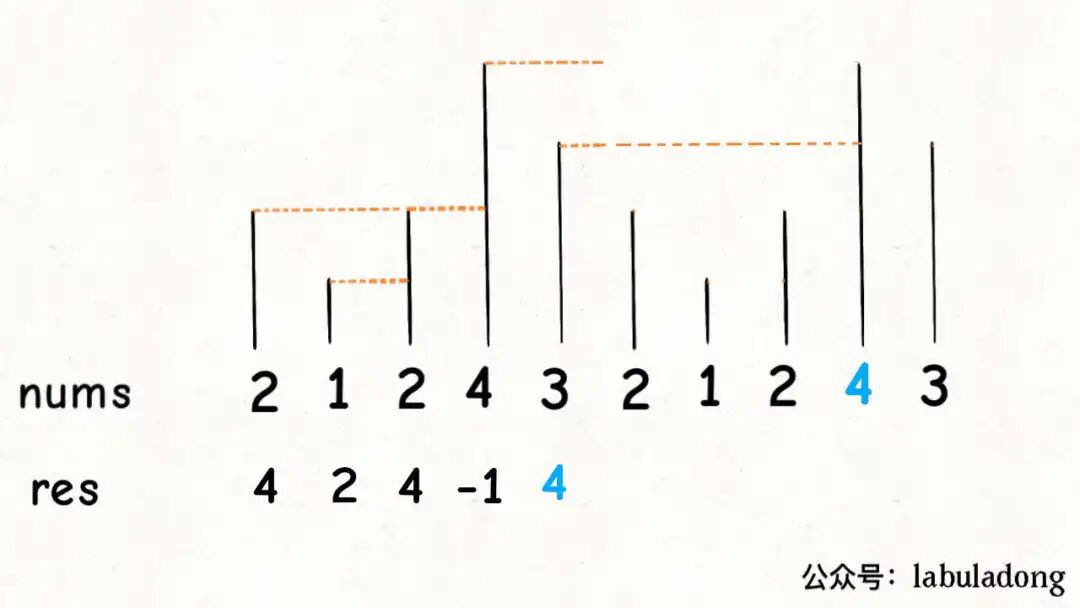## Next Greater Number

``````vector<int> nextGreaterElement(vector<int>& nums);
````````````vector<int> nextGreaterElement(vector<int>& nums) {
vector<int> res(nums.size()); // 存放答案的数组
stack<int> s;
// 倒着往栈里放
for (int i = nums.size() - 1; i >= 0; i--) {
// 判定个子高矮
while (!s.empty() && s.top() <= nums[i]) {
// 矮个起开，反正也被挡着了。（从后往前遍历，top就是后面的矮个，nums[i]是前面的高个）
s.pop();
}
// nums[i] 身后的 next great number
res[i] = s.empty() ? -1 : s.top();
//
s.push(nums[i]);
}
return res;
}
``````

## 问题变形

``````vector<int> dailyTemperatures(vector<int>& T) {
vector<int> res(T.size());
// 这里放元素索引，而不是元素
stack<int> s;
/* 单调栈模板 */
for (int i = T.size() - 1; i >= 0; i--) {
while (!s.empty() && T[s.top()] <= T[i]) {
s.pop();
}
// 得到索引间距
res[i] = s.empty() ? 0 : (s.top() - i);
// 将索引入栈，而不是元素
s.push(i);
}
return res;
}
``````

## 如何处理环形数组``````vector<int> nextGreaterElements(vector<int>& nums) {
int n = nums.size();
vector<int> res(n);
stack<int> s;
// 假装这个数组长度翻倍了
for (int i = 2 * n - 1; i >= 0; i--) {
// 索引要求模，其他的和模板一样
while (!s.empty() && s.top() <= nums[i % n])
s.pop();
res[i % n] = s.empty() ? -1 : s.top();
s.push(nums[i % n]);
}
return res;
}
``````